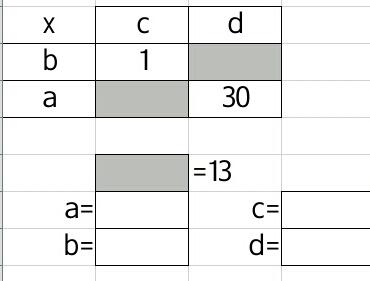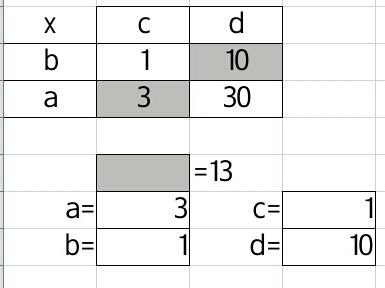To an experienced mathematician, factorising a quadratic (with real roots) is a little number puzzle, into which the algebraic terms fall gently into place.

To a secondary (high) school student of middling ability they can be algebraic torments conjured from the darkest recesses of a fevered genius’ imagination. Impossible!

This year I have introduced factorising with no mention of algebra, equations, solving or factorising. My class are at least C grade students who have convinced themselves they are no good at algebra. We started by considering this puzzle:

What values of a, b, c &d make this multiplication grid true?

bc = 1
ac + bd = 13The solution is fairly straightforward:Once they got the hang of this kind of puzzle, I compared it to the grid method for expanding double brackets. I asked them to think about what the brackets could be if I gave them the values for bc, bd, ac & ad.

Finally the stabilisers were taken off. I asked them what the brackets were if I gave an algebraic form of the number puzzle. First we considered:

bc = X squared co-efficient
ac + bd = X co-efficient